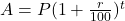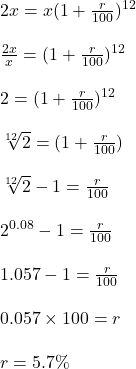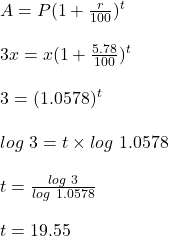## according to a survey, the population of a city doubled in 12 years. The annual rate of increase of the population of this city is appr

Question

according to a survey, the population of a city doubled in 12 years.
The annual rate of increase of the population of this city is approximately _____. The population will grow to three times its current size in approximately ______.

First box of answers: 2.50, 5.78, 12.0, 50.0

Second box of answers: 18, 19, 23, 24.

in progress 0
2 months 2021-09-05T07:30:18+00:00 2 Answers 0 views 0

5.78 and 18 respectively

5.78

19

Step-by-step explanation:

Let original population be, P = x

Growth in 12 years, A = 2x

Rate be = r

Time = 12years

Find the rate :The annual rate of increase of the population of this city is approximately 5.78.

Find  time in which the population becomes 3 times.

That is A = 3x

P = x

R= 5.78%The population will grow to three times its current size in approximately 19years .